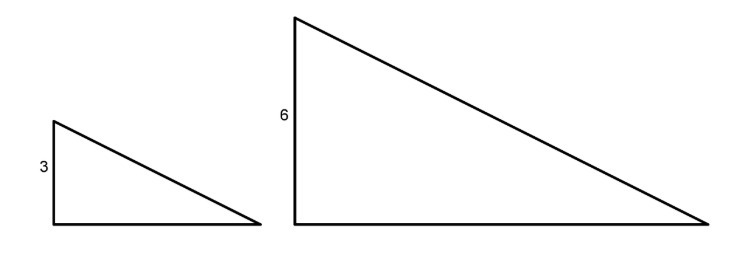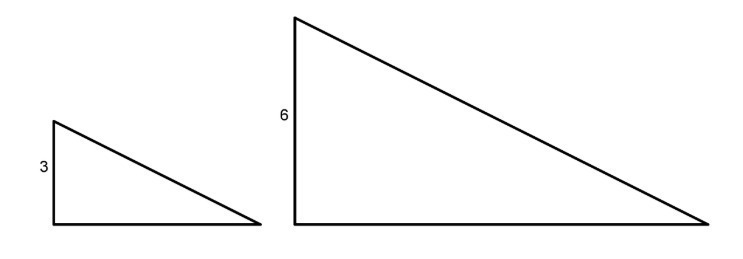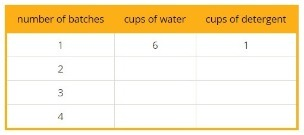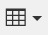PSA6SP4 - Practice Problems---7.1 Lesson 12: Units in Scale Drawings (7.G.A.1)
Part A)

The Empire State Building in New York City is about 1,450 feet high (including the antenna at the top) and 400 feet wide. Andre wants to make a scale drawing of the front view of the Empire State Building on an 1/2-inch-by-11-inch piece of paper. Select a scale that you think is the most appropriate for the scale drawing.

Select one:
Part B)

Elena finds that the area of a house on a scale drawing is 25 square inches. The actual area of the house is 2,025 square feet. What is the scale of the drawing?

Complete the following scale:

1 inch to ___ feet

Type your answer below as a number (example: 5, 3.1, 4 1/2, or 3/2):

Which of these scales are equivalent to 3 cm to 4 km? Select all that apply. Recall that 1 inch is 2.54 centimeters.

Select all that apply:
Part A)

These two triangles are scaled copies of one another. The area of the smaller triangle is 9 square units. What is the area of the larger triangle?Type your answer below as a number (example: 5, 3.1, 4 1/2, or 3/2):
Part B)

These two triangles are scaled copies of one another. The area of the smaller triangle is 9 square units. What is the area of the larger triangle? Explain or show how you know.Explain or show how you know.

Part A)

Water costs $1.25 per bottle. At this rate, what is the cost of 10 bottles? Do not include units ($) in your answer.

Type your answer below as a number (example: 5, 3.1, 4 1/2, or 3/2):
Part B)

What is the cost of 20 bottles?

Do not include units ($) in your answer. Type your answer below as a number (example: 5, 3.1, 4 1/2, or 3/2): Part C) What is the cost of 50 bottles? Do not include units ($) in your answer.

Type your answer below as a number (example: 5, 3.1, 4 1/2, or 3/2):
Part A)

The first row of the table shows the amount of dish detergent and water needed to make a soap solution.

Complete the table for 2, 3, and 4 batches.Create and fill in the table using the table buttonPart B)

How much water is needed for 8 batches?

Type your answer below as a number (example: 5, 3.1, 4 1/2, or 3/2):
Part C)

How much detergent is needed for 8 batches?